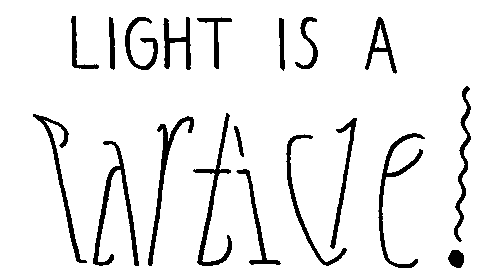## Wave-Particle Duality of light

Light behaves like a wave

• Diffraction
• Interference
• Polarize

Light behaves like a particle when it strikes matter

• Momentum is transferred
• Photoelectric effect- electrons are kicked out

(If you watch any more than 5:30, your brain will hurt)

## Photoelectric effect

1905 Einstein
What he actually got the Nobel Prize for.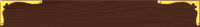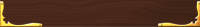Classification of matter## Worksheets

Photons

Photoelectric

Photoelectric Effect 2A = ammeter which measures photocurrent (# of e- moving)
Volts supply the attraction of repulsion of emitted electrons.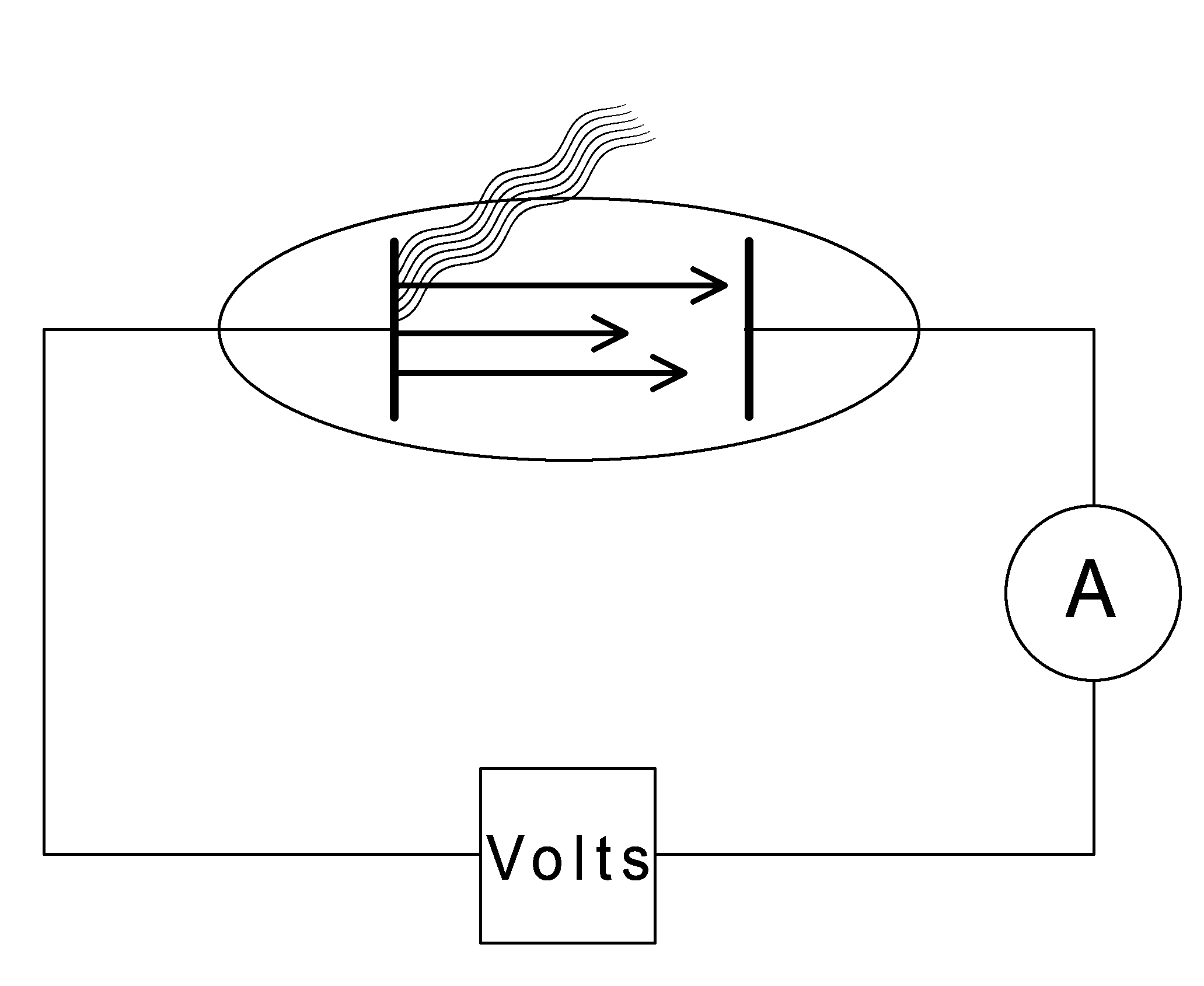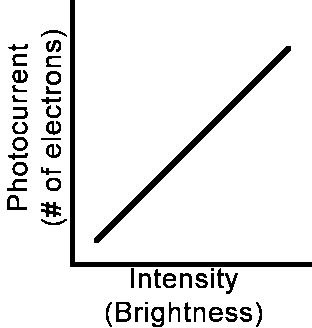The results of the experiment showed that as the light got brighter more electrons were emitted.

Photoelectric Effect Simulation:

To measure the KE of each electron:

1. Turn on voltage so that the plate on right repels electrons.
2. Adjust voltage (V0) so that the photocurrent just stops.
3. V0 is the “stopping potential”

By controlling the voltage he knew how much work/charge (V=W/q)
And therefore knew the energy (KE) of the electrons: KE = W
The kinetic energy of the electrons does not depend on intensity. (Although brighter light made more electrons come off of the plate, they did not travel faster- their speed remained the same.)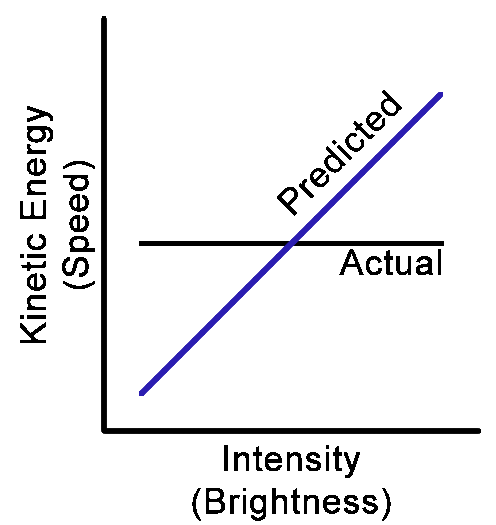This graph shows that the speed of the electrons does not change no matter how bright the light was. Brightness makes more or less electrons come off of the metal but they all come of at the same average speed.   The slope of this graph (KE vs f) is known as Plank's constant (h) which is used to calculate the amount of energy of a certain frequency of light. h = 6.63 x 10-34 J•s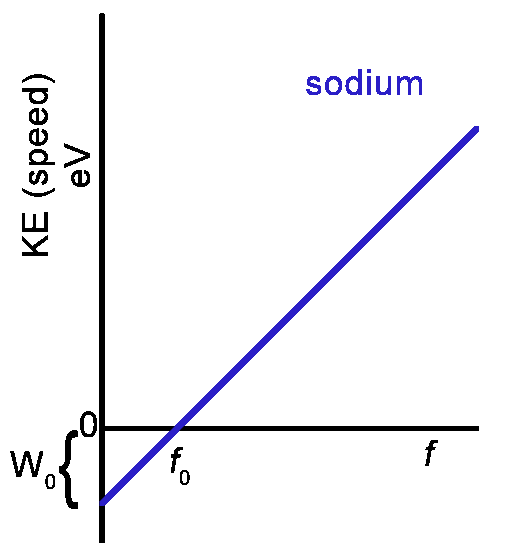Some colors (f) do not emit electrons while others do emit electrons. Higher energy f made e- move faster (more KE) The frequency of light (color) was changed. This had the effect of changing the speed of the electrons while the amount of electrons stayed the same (from the brightness). Low frequency (towards the red end) waves have low energy. High frequency waves (towards the blue end) have higher energy. As the frequency of light was lowered (more red) the electrons moved slower until eventually they stopped all together and no more were emitted. The frequency of light that caused the electrons to stop is called the “threshold frequency”. Below the threshold: no electrons emitted. Above the threshold: electrons emitted. Way above the threshold: electrons move faster. Below the threshold frequency (f0, the energy of the light is used to lift the electron away from the attractive force nucleus. If the energy is not high enough, the electron will fall back to its ground state. Once it receives enough energy, it will lift far enough to escape. The amount of energy required to lift the electron away from the nucleus is called the “Work Function” (W0) . Any leftover energy from the light is used to make the electron go faster. This chart shows that the speed of the electrons does not change no matter how bright the light was. Brightness makes more or less electrons come off of the metal but they all come of at the same average speed. (*More details later on how the electrons absorb certain amounts of the energy from light.) In this example, a photon with an energy of 10 electron Volts (eV) strikes an electron in the ground state. The photon supplies the electron with energy causing it to rise to a higher orbit. In this case, it only needs 8eV to rise to the highest possible orbit. The remaining 2eV is used to kick the electron out of the atom and give it some speed. Ephoton - W0 = KEelectron 10eV - 8eV = 2eV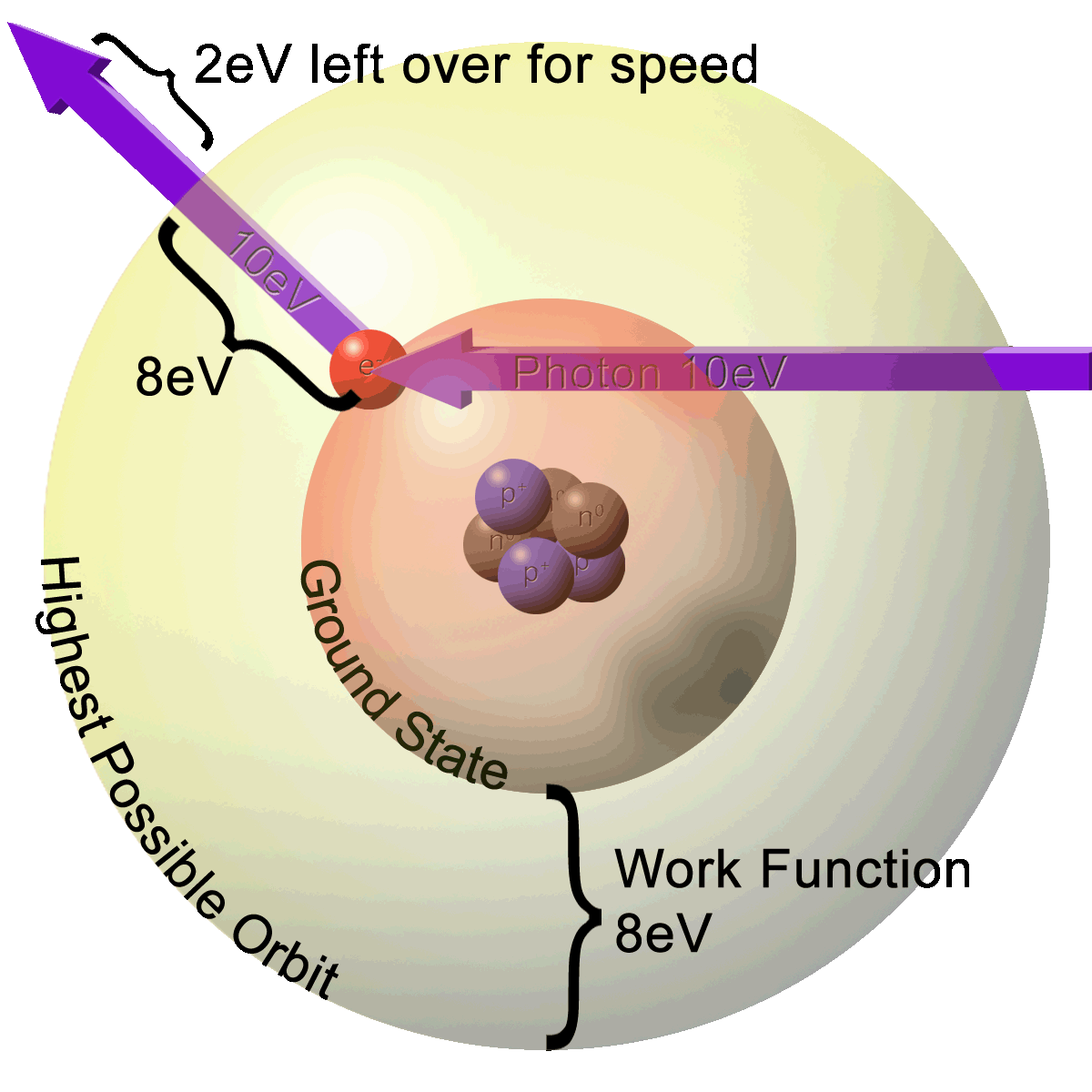(Image drawn by Phil Medina)
 To find the energy of the Work Function (W0) you need to find the energy of a photon of the threshold frequency's (f0) light. W0 = hf0 h = Plank's constant (6.63 x 10-34Js ) If f0 = 5.75 x 1012Hz (green light) then: W0 = hf0 W0 = (6.63 x 10-34Js)(5.75 x 1012Hz) W0 = 3.81 x 10-19J

 To Sum up: • An atom likes to hold its electrons. It will take some energy just to release it. • Red has a low f, low energy, longer λ • Blue has a high f, higher energy, smaller λ • The lowest f that will have enough energy to emit an electron is the “threshold frequency” (f0) • Zero energy on the graph means that the electron has just enough energy to be released from the atom, but none left for KE. • W0 (work function) is the amount of energy needed to release the electron. • E ∝ f: higher frequency light has more energetic photons. • Slope of KE vs f is Planck’s constant (h) • E = hf • h = 6.63 x 10-34J•s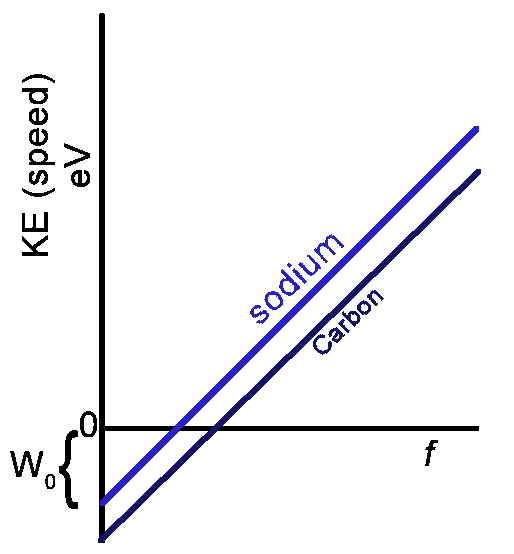• All atoms have the same slope (h) but different work functions. • In this case Carbon has a bigger work function (difference between 0 and y-intercept) • Sodium needs less energy to release an atom than Carbon. – Ex: Sodium might release an e- in red light or higher where Carbon will need blue light.

## Compton Effect:

Proof that light particles have momentum- they act like particles!

 Electron has energy: KE = ½mv2 before the collision the electron is stationary KE = 0 X-ray has energy: E = hf   After collision: Conservation of energy KEelectron goes up (it moves) E of x-ray goes down with a lower f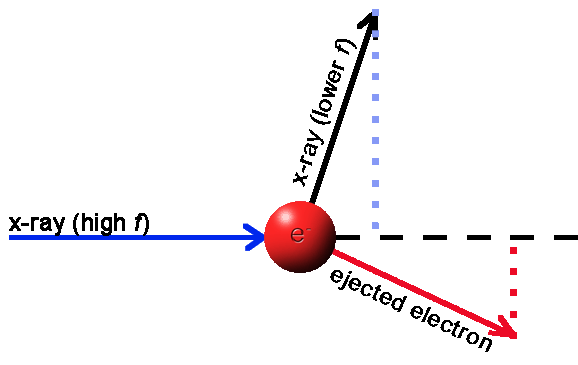(Image drawn by Phil Medina) This experiment proved that photons (particles of light) have momentum. The amount of momentum is proportional to the energy (frequency) of the photon. Law of conservation of momentum still works on the quantum level:   Before: electron has no KE and photon has energy from its frequency After: the electron has gained energy and the photon lost the same amount Moral of the story: waves act like particles.

## DeBroglie

Mathematically proved that matter has wave-like characteristics.

 Givens: p: momentum (p = mv from impulse/momentum chapter) E: energy c: speed of light h: Plank's constant v: velocity (in the case of photons v = speed of light) f: frequency λ: wavelength m: mass p = E/c (derived from Compton- the derivation is not shown here) p = momentum = mv E = hf (photoelectric) v = f λ (therefore c = fλ ) ...and DeBroglie's derivation: p = momentum = mv p = E/c substitute hf for E and h/λ for c gives: hf/fλ the f's cancel out leaving: h/λ So, p = mv becomes: p = h/λ Switched around gives: λ = h/p substitute mv for p gives: λ = h/mv Therefore: λmatter = h/mv Moral of the story: matter behaves like a wave. *Wavelength is inversely proportional to momentum (mass). Which translates into: "The bigger the mass the smaller the wavelength. The smaller the mass the longer the wavelength."

## Matter Waves

 Everything has both a particle nature and a wave nature. Massive are more particle-like and less wave-like. Less massive are more wave and less particle. Therefore: λmatter = h/mv Electrons have wave properties where: λ ~ 10-12m which is about the λ of an x-ray. As waves electrons can Diffract Interfere be used as electron microscopes

## JJ Thomson's "Plum Pudding"

• Approximated the size of an atom
• He surmised that atoms were neutral and electrons can be ejected so there must be positives
• Pudding is a smear of positives
• Electrons are sprinkled.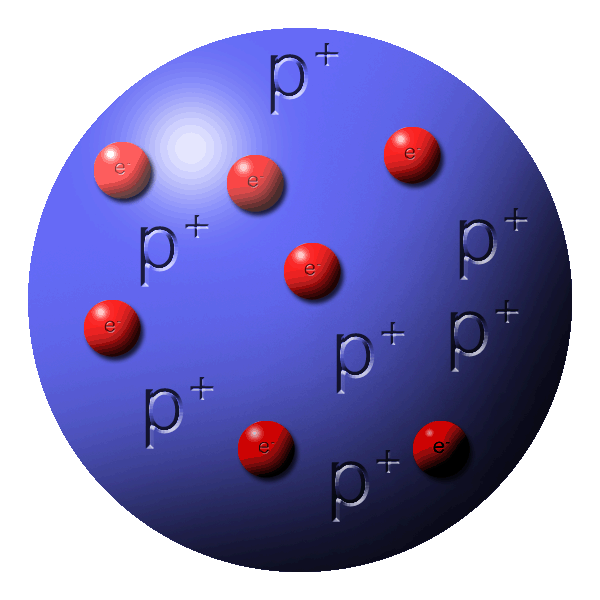(Image drawn by Phil Medina)

## Ernest Rutherford model

Very thin foil of gold was blasted with alpha particles (Helium Nucleus: 2+ and 20)

• Most particles pass straight through and glow on a phosphorous screen.
• Small percent bounced in random directions.
• Conclusions:
• Atom is mostly empty space
• Nucleus was very dense and positively charged

According to this model the atom should emit a continuous spectrum of light yet it only makes specific colors.

## Smashing atoms

• Nucleus is protons and neutrons
• Protons hate each other (electrostatic force repels) but are held together with the strong force (it must be strong to overcome the electrostatic)
• The strong force is a VERY short distance force.
• When blasted, the protons are spread far enough to overcome the strong force and the electrostatic takes over.
• The atom explodes- fission (fission is division)

## Nuclear processes

(Please sound like you know what you're saying. “nuclear is pronounced “new-clear” or “new-klee-are”. Do not sound like George W by saying “new-cue-lar”)

Fission (division) splitting atoms

• Relatively esy to do
• Is the process for most nuclear bombs

Bomb Compilation:

Underground detonation

Scars from underground nuclear tests

View Larger Map
37.111529, -116.0459

## Controlling Fission

When radioactive atoms decay, they send out a few particles. If these particles hit other nuclei, it causes them to split and continue the process into a “chain reaction”. As the atoms are brought closer, the chances of hitting another nucleus increases and the chain reaction happens faster.

• A slow controlled chain reaction is a nuclear reactor
• The rate of the chain reaction is controlled with rods of carbon inserted between fuel rods to absorb the decay particles. When the rods are inserted, the reaction happens slower. When removed, the reaction is faster.
• A fast, out-of-control reaction is a nuclear bomb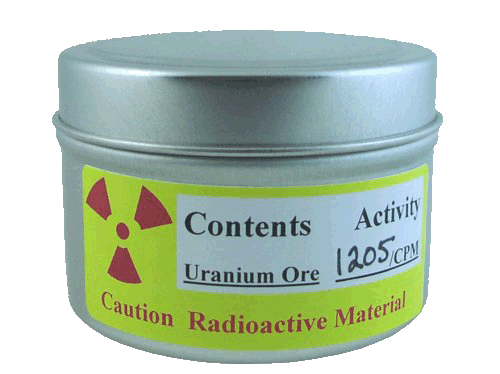http://www.amazon.com/Images-SI-Inc-Uranium-Ore/dp/B000796XXM

## Fusion

• “to fuse, or weld, things together"
• Putting small atoms to make larger ones
• Releases much more energy
• Requires much higher temperatures and pressures

## Annihilation

Matter and antimatter (antimatter will be explained more in classification of matter below) combine to completely obliterate each other and turn into pure energy.

## E = mc2

Einstein’s famous equation. Although this is probably the most famous equation in the world, very few people understand what it actually means. It tells you how much energy is released when matter is destroyed and converted into energy.

• E = energy
• m = mass
• c = the speed of light (299,792,458 m/s )

The equation tells us that matter can be converted into energy and energy can be converted into matter.

During nuclear processes any mass lost will be converted according to E=mc2.

• For large masses (on the order of kg) use E=mc2 to convert from kg to energy in Joules
• For atomic scales:
• Convert universal mass units (μ) into electron volts (eV)
• 1μ is about the mass of a proton
• 1μ = 9.31 x 102MeV
• Front page of Reference tables

Mass is lost when protons and neutrons are combined to make Helium. The lost mass is converted into energy
Helium is made of 2 protons and 2 neutrons
1p+ = 1.007 276μ
1 neutron = 1.008 665μ

 1.007 276μ 1.007 276μ 1.008 665μ 1.008 665μ = 4.031 883μ

While Helium is 4.002 602μ

 4.031 883μ -4.002 602μ 0.029281μ

There is a missing mass of 0.029281μ
0.029281μ = 0.27260611 x 102Mev of energy that is released during the fusion of Helium.

## Neils Bohr

Absorption spectrum

• White light shines into a gas.
• Some of the light that passes through will be used by electrons.
• That light does not pass through the gas and shows up as a dark line on the other side when viewed through a prism.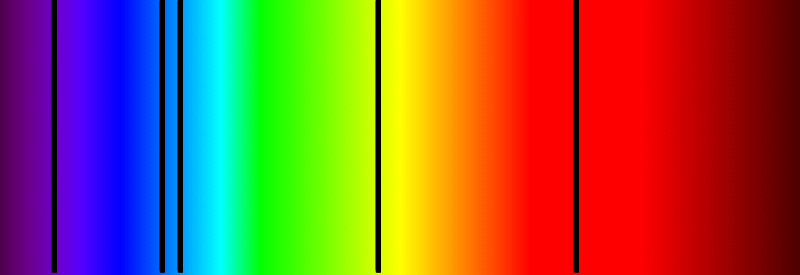Emission spectrum

• Gas is heated and electrons will absorb the energy and begin to glow.
• The glow shows up as colored lines when viewed through a prism.Absorption and emission lines of an element are at exactly the same frequencies as each other.• Electrons go around the nucleus in specific orbits.
• Electrons jumping from orbit to orbit absorb or release energy- in specific amounts called "quanta".
• When an electron is in the first level it is grounded.
• It will get excited and jump up to the next stable orbit only when it absorbs just the right amount of energy.
• Electrons can only jump into specific orbits numbered 1, 2, 3 etc.
• Each level gets only specific amounts of energy.
• No fractional jumping (ex 2.3 is not allowed)
• Each level is called a quantum number.
• The energy values for each level are specific only for one element.

## Conversion Practice:

Change 2.1eV into Joules
Change .26eV into Joules

Then convert those energies into frequencies having that amount of energy.

## Energy levels

When an electron accepts a photon, it will jump up to a higher level (higher orbit).

Start at 2:41

Rather than climbing steadily up and out of an atom, electrons jump up steps.
Each step is a level and there are only set values for each level.

## Energy involved in jumping orbits:

• -13.6eV is the W0 specific for hydrogen.
• (It is a negative number because you need to give it 13.6eV just to get it UP to the zero level where it could escape.)
• There are energy levels under the W0 where the electrons jump up and drop down but stay attached to the atom.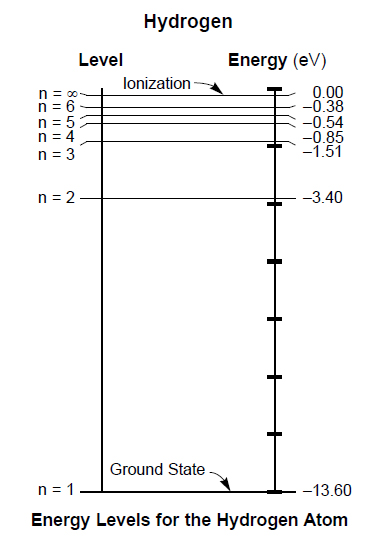## Quantum Theory (quantum- quantity get it?)

Photons have only set amounts (packets) of energy depending on the frequency of the light.
Each packet is a "quantum".
Planck’s Constant (h = 3.63 x 10-34Js) tells how much energy each quantum has: E = hf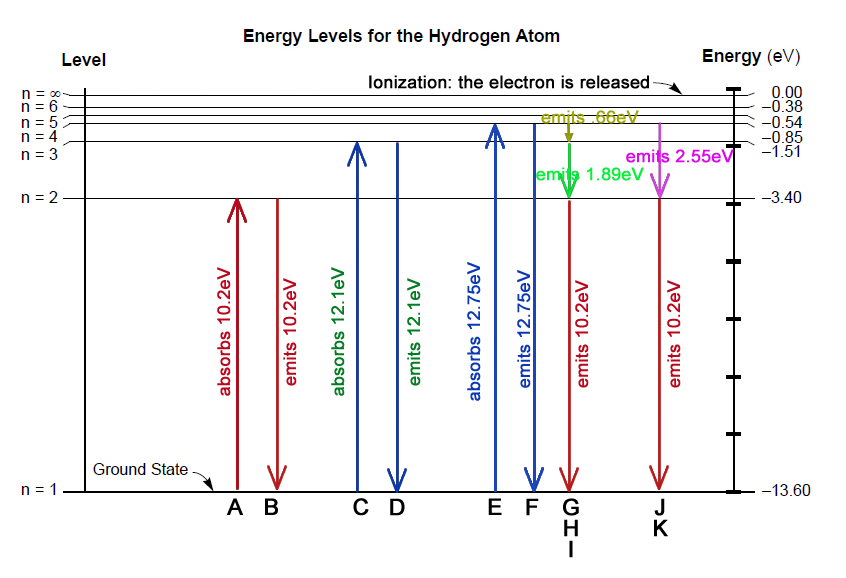Each possible orbit for an electron in Hydrogen is shown in this chart numbered 1 - 6 and ∞ 1 is the ground state. ∞ is the point at which the electron escapes and the atom becomes ionized. It requires 13.2eV to just escape the atom which is called the work function (W0). To reach up to the work function it will require 13.2eV. When the electron is in the ground state it requires 13.2eV to reach this zero point (W0). This is shown as a negative number. A: The electron will require a photon with an energy of 10.2eV to get from the ground state (-13.2eV) up to n = 2 which has an energy of 3.4eV B: The electron releases a photon of 10.2eV to return to the ground state. C: To go from the ground state (n = 1) up to n = 3 it will require a photon with an energy of 12.1eV (13.6eV – 1.51eV). D: To drop down from level n = 3 to the ground state. The electron will release 12.1eV E: To reach n = 4 (be careful of the curved label lines showing the levels and energies) the electron will need 12.75eV (13.6eV - .85eV). F: The electron releases 12.75eV to get back to the ground state. G,H,I: The electron can stop at any stable orbits is passes on its way back to the ground level, releasing the required amount of energy for each. G: To drop from n = 4 to n = 3 it releases .66eV H: To drop from n = 3 to n = 2 it releases 1.89eV I: to drop from n = 2 to n = 0 (ground state) it releases its final 10.2eV J, K: The electron drops from n = 4 to n = 2, thus skipping n = 3 (because it felt like it!) releasing a total of energy with the difference between those two levels: 2.55eV (3.4eV - .85eV). It drops the remaining level to release 10.2eV which we have seen before (B & I)

## Schrödinger’s Cloud Model

 Electrons do not have an exact orbit to follow as in Bohr’s model but rather have regions in which they can be found.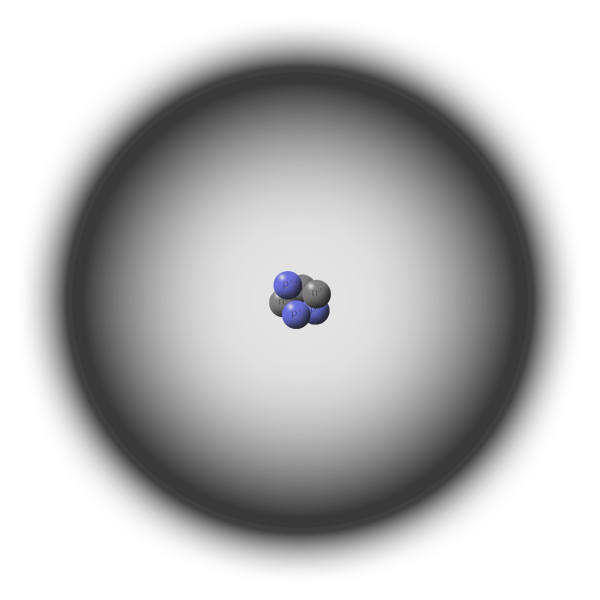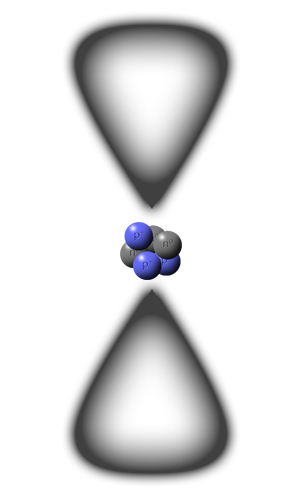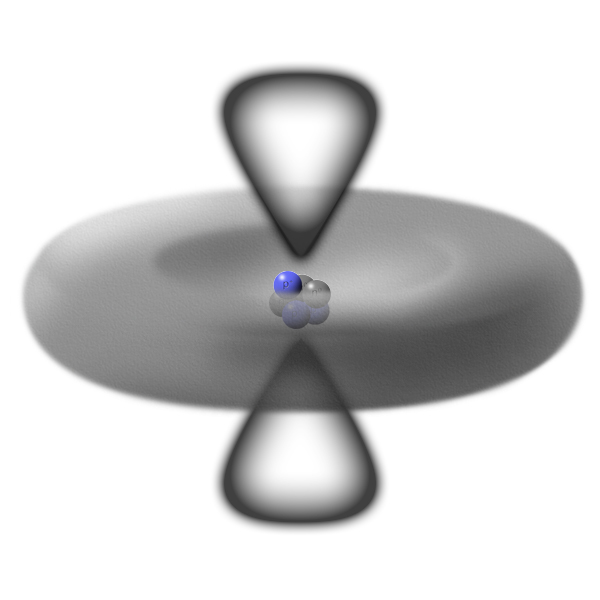Schrödinger’s Cat No mention of Schrödinger would be complete without mentioning his cat. Essentially it is a thought experiment where there is a cat locked in a box with some sort of lethal device (bomb, poison, electrocution or whatever your sick imagination can devise) There is a 50/50 chance that the cat has already been killed by the device. Until you open the box, you do not know if the cat is dead or alive. Until the cat’s state is observed you have to deal with the possibility of both simultaneously because either case can exist. Only by observing the cat do you confirm the health of the cat. In quantum physics, there are probabilities that something will happen. For example, the electrons in an atom can exist anywhere within the “cloud of probability” so it must be dealt with as if it was anywhere within that cloud. Only when the electron is actually observed do you know its exact location yet your theories must account for all of the other possabilities.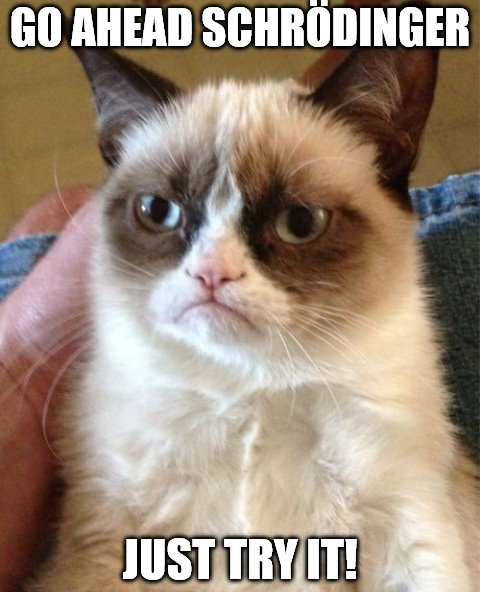# Classification of Matter

###### Note: The information below is targeted to an introductory High School Physics class. Explanations do not go into depth and are simplified to meet with the curriculum of this course.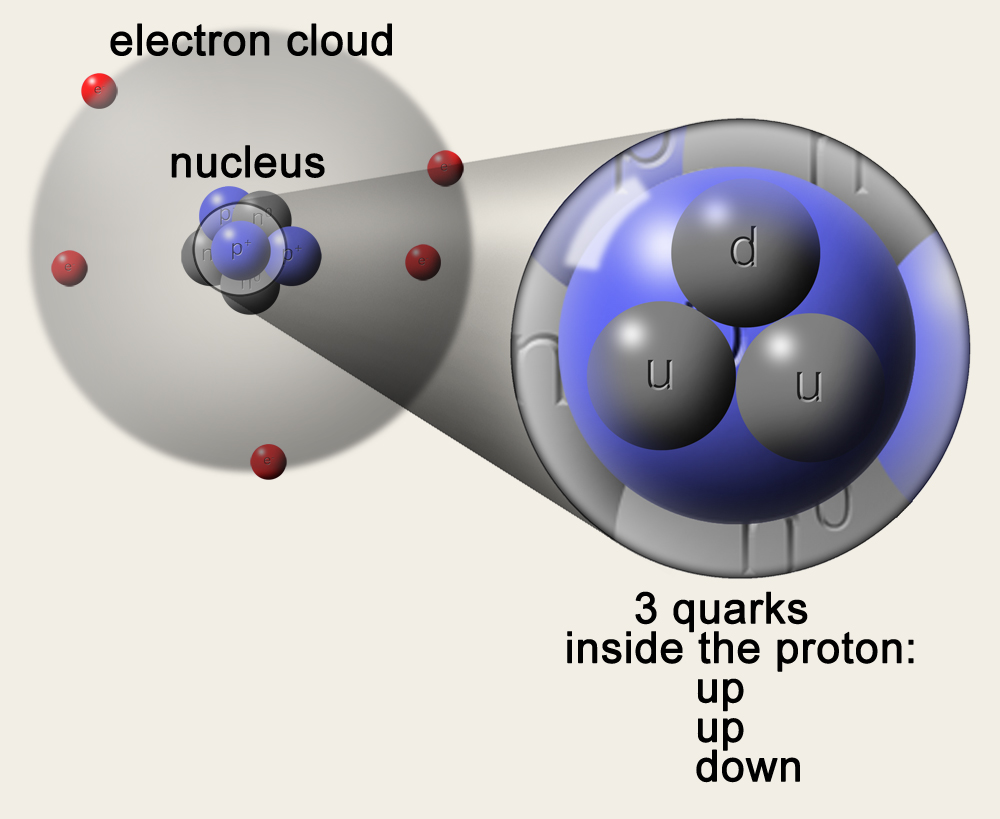Matter can be reduced into atoms. Atoms can be reduced into protons, neutrons and electrons. Electrons cannot be reduced into anything smaller. In this context, they are called “Leptons”. Protons and Neutrons can be reduced into Quarks. In this context, they are called “Hadrons”. Protons and Neutrons are made of three quarks.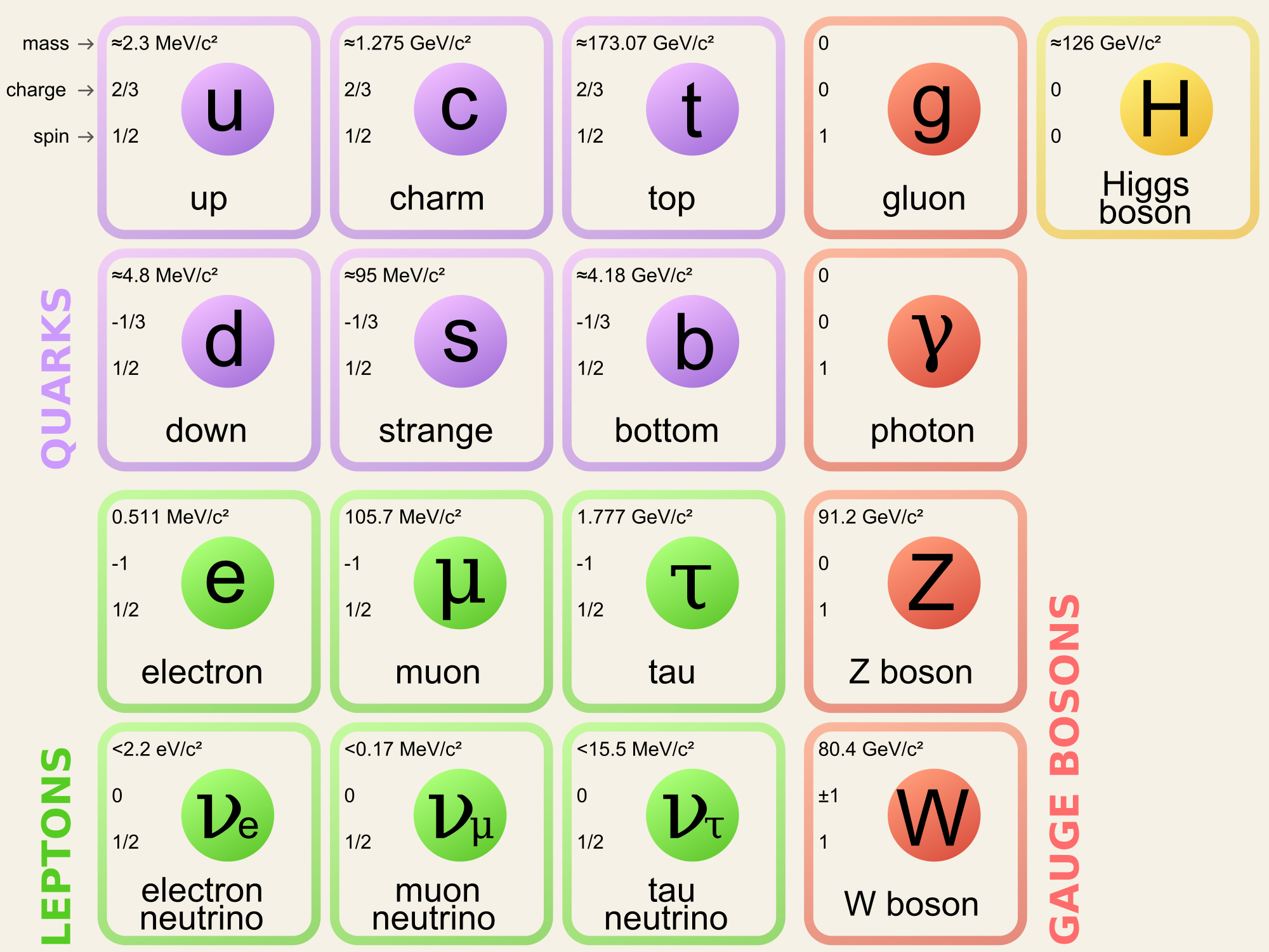(Graphic from wikipedia) Classification of Matter Chart AKA "The Standard Model" The kind of matter that makes up the stuff you and I interact with is made up of particles in the first column: up, down, electron. The second column (topped with charm and top quarks) shows particles that existed in the second (roughly) after the Big Bang and only currently exist in particle accelerators. The last two columns list the known bosons. (Bosons are not part of the curriculum of my course and will not be discussed here. Of course, you can find more information on these on wikipedia here: elementary particle ) For each particle there is a corresponding antiparticle with a charge opposite that of its associated particle which will be discussed in more detail below.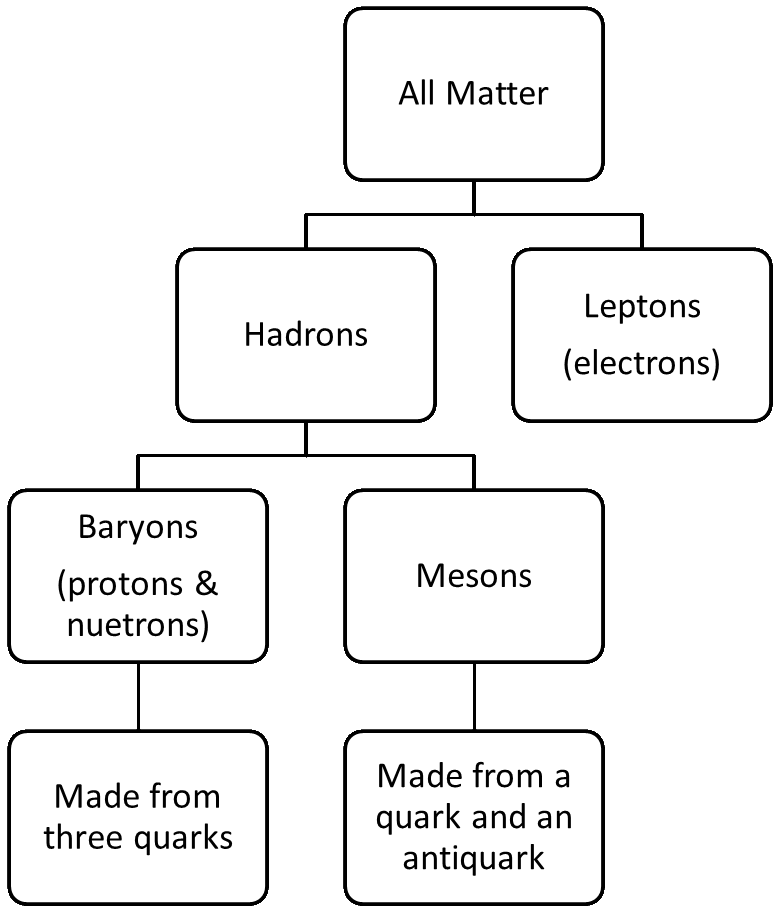Matter is broken down into Leptons and Hadrons Leptons = electrons and cannot be reduced. Hadrons can be broken into smaller parts: Baryons are made of three parts and can be either protons or neutrons. Mesons are made of two parts and make "other things". Baryons come in three quarks that add up to the charge of that particle: Proton +1 = uud (+2/3, +2/3, -1/3) Neutron 0 = udd (+2/3, -1/3, -1/3 ) u = an up quark with a value of +2/3 d = a down quark with a value of -1/3 Antimatter Antimatter is exactly the same as regular matter except its charge has been reversed. When a particle and its corresponding antiparticle touch, they annihilate and release energy according to E = mc2. electron... antielectron (AKA positron) proton... antiproton neutron... antineutron

## The Higgs Boson

The Higgs Boson is a recent discovery, or rather, confirmation or a predicted particle. The bosons are called “force carriers” and it really gets strange when you’re talking about bosons (yes, even stranger than “charm”, “top”, “bottom” and indeed “strange” particles). Bosons are exchanged between two particles and the exchange produces a force. The Higgs gives a particle its mass- but never mind all of that. The real importance of the discovery of Higgs is that it is a confirmation that the Standard Model. The Standard Model predicted the existence of a particle with all of the properties of what will be called the Higgs Boson. The Higgs was finally produced and observed in a particle accelerator. This discovery was a confirmation that The Standard Model is, so far, an accurate explanation of how matter and forces work.

A description of the Higgs Boson:
http://www.phdcomics.com/comics/archive.php?comicid=1489

Answers to the conversion problem way above:
5.2 x 1019Hz (X-Ray)
6.27 x 1013Hz (Infrared)
Back to the conversions practice.Medina On-Line
Since 1994
Email: mrsciguy@optonline.net